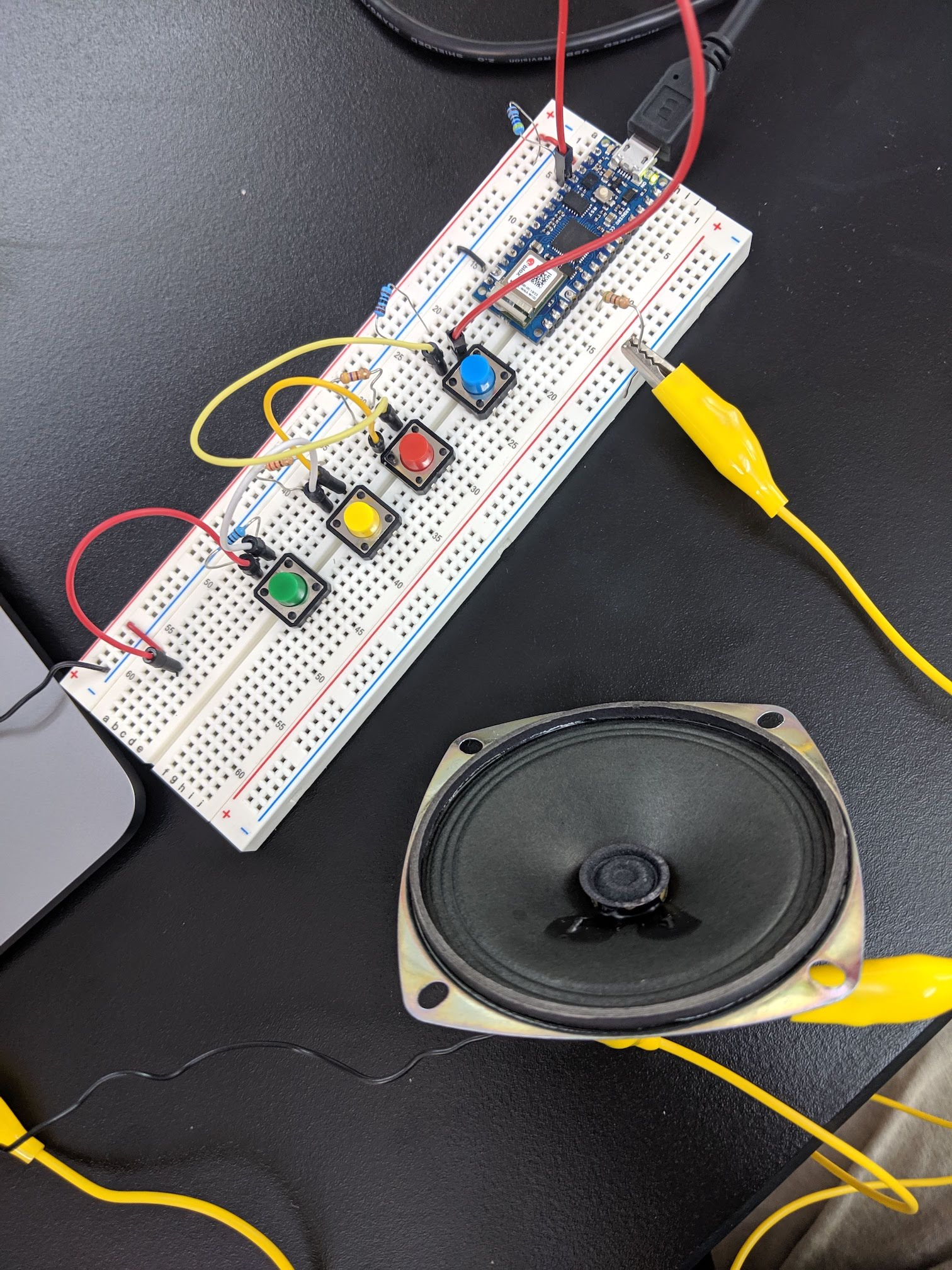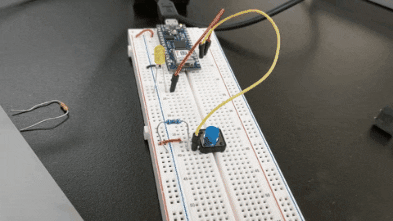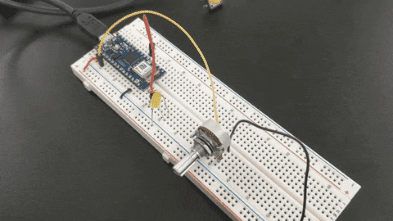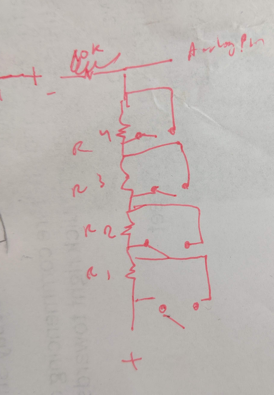# Physical Computing - Push Button Synthesizer# Basic I/O with the Arduino Nano 33 IOT

I started with wiring a push button and LED - the simplest form of Arduino I/O. The LED is connected to the D3 pin and the push button is wired to the D2 pin. I added a small resistor to the LED to ensure the 3.3V from the board doesn’t burn it out, and a larger 10K resistor from the D2 input pin to ground to ensure the pin has a stable connection to ground while still diverting power to the pin (instead of causing it to go straight to ground).

``````void setup() {
pinMode(2, INPUT);
pinMode(3, OUTPUT);
}

void loop() {
}
``````This setup doesn’t preserve state (the LED is only lit while the button is held).

Next, I connected a potentiometer to the A0 input pin to dim the LED as the resistance changes (controlled by the pot).

``````void setup() {
pinMode(3, OUTPUT);
}

void loop() {
}
``````# Pushbutton Synthesizer

To challenge myself I set the goal of making a basic synthesizer. For bonus points, I wanted to control the notes by wiring several switches into a single analog input (instead of simply reading from multiple digital inputs). Although ultimately I only use four switches, this technique could potentially be useful in a situation where I want to process a ton of inputs without having to get multiple microprocessors. I came up with a circuit design where each of the four switches opens a path to skip an associated resistor. Each of the four resistors is approximately twice the resistance of the last - this way the sum of any group will be unique. This means that each arrangement of the four switches would translate to a specific resistance and specific analog in value, allowing for fifteen individual pitches.I had a lot of trouble getting the tone() command to work properly, until I realized that I needed to only call it when the frequency value changed (instead of repeatedly calling it with the same arguments every iteration of loop()). To accommodate this I added a simple state-tracking mechanism to only trigger if the last recorded frequency was different from before. I also added smoothing, averaging over the last 16 inputs in order to calm the signal which was quite noisy at the expense of barely noticeable latency.

``````// Store a circular buffer of the last 16 reads
// which we can then average to smooth the input signal
const int n = 16;
int values[n];
int r_index = 0;
float lastfreq = 0;

int thresholds = {
570,
585,
600,
625,
647,
669,
690,
720,
745,
775,
801,
850,
880,
925,
960
};

float notes = {
261.63, // D4
277.18,
293.66,
311.13,
329.63,
349.23,
369.99,
392.00,
415.30,
440.00, // A4
466.16,
493.88,
523.25,
554.37,
587.33
};

void setup() {
for (int i = 0; i < n; i++) {
values[i] = 0;
}
}

void loop() {
values[r_index] = val;
r_index++;
if (r_index >= n) {
r_index = 0;
}
int sum = 0;
for(int i = 0; i < n; i++) {
sum += values[i];
}

int newval = sum / n;
float freq = 0;
for(int i = 14; i >= 0; i--) {
if (newval > thresholds[i]) {
freq = notes[i];
break;
}
}
if (freq == 0 && lastfreq != 0) {
noTone(12);
lastfreq = freq;
} else if (freq != lastfreq) {
tone(12, freq);
lastfreq = freq;
}
delay(1);
}
``````